geom_mlb_logos() and geom_mlb_scoreboard_logos() are used to plot MLB team and league logos instead of points in a ggplot. It requires x, y aesthetics as well as a valid MLB team abbreviation. The latter can be checked with valid_team_names() but is also cleaned before being plotted.

## Usage

geom_mlb_logos(
mapping = NULL,
data = NULL,
stat = "identity",
position = "identity",
...,
nudge_x = 0,
nudge_y = 0,
na.rm = FALSE,
show.legend = FALSE,
inherit.aes = TRUE
)

geom_mlb_scoreboard_logos(
mapping = NULL,
data = NULL,
stat = "identity",
position = "identity",
...,
nudge_x = 0,
nudge_y = 0,
na.rm = FALSE,
show.legend = FALSE,
inherit.aes = TRUE
)

## Arguments

mapping

Set of aesthetic mappings created by aes(). If specified and inherit.aes = TRUE (the default), it is combined with the default mapping at the top level of the plot. You must supply mapping if there is no plot mapping.

data

The data to be displayed in this layer. There are three options:

If NULL, the default, the data is inherited from the plot data as specified in the call to ggplot().

A data.frame, or other object, will override the plot data. All objects will be fortified to produce a data frame. See fortify() for which variables will be created.

A function will be called with a single argument, the plot data. The return value must be a data.frame, and will be used as the layer data. A function can be created from a formula (e.g. ~ head(.x, 10)).

stat

The statistical transformation to use on the data for this layer, either as a ggproto Geom subclass or as a string naming the stat stripped of the stat_ prefix (e.g. "count" rather than "stat_count")

position

Position adjustment, either as a string naming the adjustment (e.g. "jitter" to use position_jitter), or the result of a call to a position adjustment function. Use the latter if you need to change the settings of the adjustment.

...

Other arguments passed on to ggplot2::layer(). These are often aesthetics, used to set an aesthetic to a fixed value. See the below section "Aesthetics" for a full list of possible arguments.

nudge_x, nudge_y

Horizontal and vertical adjustment to nudge labels by. Useful for offsetting text from points, particularly on discrete scales. Cannot be jointly specified with position.

na.rm

If FALSE, the default, missing values are removed with a warning. If TRUE, missing values are silently removed.

show.legend

logical. Should this layer be included in the legends? NA, the default, includes if any aesthetics are mapped. FALSE never includes, and TRUE always includes. It can also be a named logical vector to finely select the aesthetics to display.

inherit.aes

If FALSE, overrides the default aesthetics, rather than combining with them. This is most useful for helper functions that define both data and aesthetics and shouldn't inherit behaviour from the default plot specification, e.g. borders().

## Value

A ggplot2 layer (ggplot2::layer()) that can be added to a plot created with ggplot2::ggplot().

## Aesthetics

geom_mlb_logos() and geom_mlb_scoreboard_logos() understand the following aesthetics (required aesthetics are in bold):

• x - The x-coordinate.

• y - The y-coordinate.

• team_abbr - The team abbreviation. Need to use Savant's abbreviation.

• alpha = NULL - The alpha channel, i.e. transparency level, as a numerical value between 0 and 1.

• colour = NULL - The image will be colourized with this colour. Use the special character "b/w" to set it to black and white. For more information on valid colour names in ggplot2 see https://ggplot2.tidyverse.org/articles/ggplot2-specs.html?q=colour#colour-and-fill

• angle = 0 - The angle of the image as a numerical value between 0° and 360°.

• hjust = 0.5 - The horizontal adjustment relative to the given x coordinate. Must be a numerical value between 0 and 1.

• vjust = 0.5 - The vertical adjustment relative to the given y coordinate. Must be a numerical value between 0 and 1.

• width = 1.0 - The desired width of the image in npc (Normalised Parent Coordinates). The default value is set to 1.0 which is big but it is necessary because all used values are computed relative to the default. A typical size is width = 0.075 (see below examples).

• height = 1.0 - The desired height of the image in npc (Normalised Parent Coordinates). The default value is set to 1.0 which is big but it is necessary because all used values are computed relative to the default. A typical size is height = 0.1 (see below examples).

## Examples

# \donttest{
library(mlbplotR)
library(ggplot2)

team_abbr <- valid_team_names()
# remove conference logos from this example
team_abbr <- team_abbr[!team_abbr %in% c("NL", "AL", "MLB")]

df <- data.frame(
a = rep(1:6, 5),
b = sort(rep(1:5, 6), decreasing = TRUE),
teams = team_abbr
)

# keep alpha == 1 for all teams including an "A"
matches <- grepl("A", team_abbr)
df$alpha <- ifelse(matches, 1, 0.2) # also set a custom fill colour for the non "A" teams df$colour <- ifelse(matches, NA, "gray")

# scatterplot of all logos
ggplot(df, aes(x = a, y = b)) +
geom_mlb_logos(aes(team_abbr = teams), width = 0.075) +
geom_label(aes(label = teams), nudge_y = -0.35, alpha = 0.5) +
theme_void()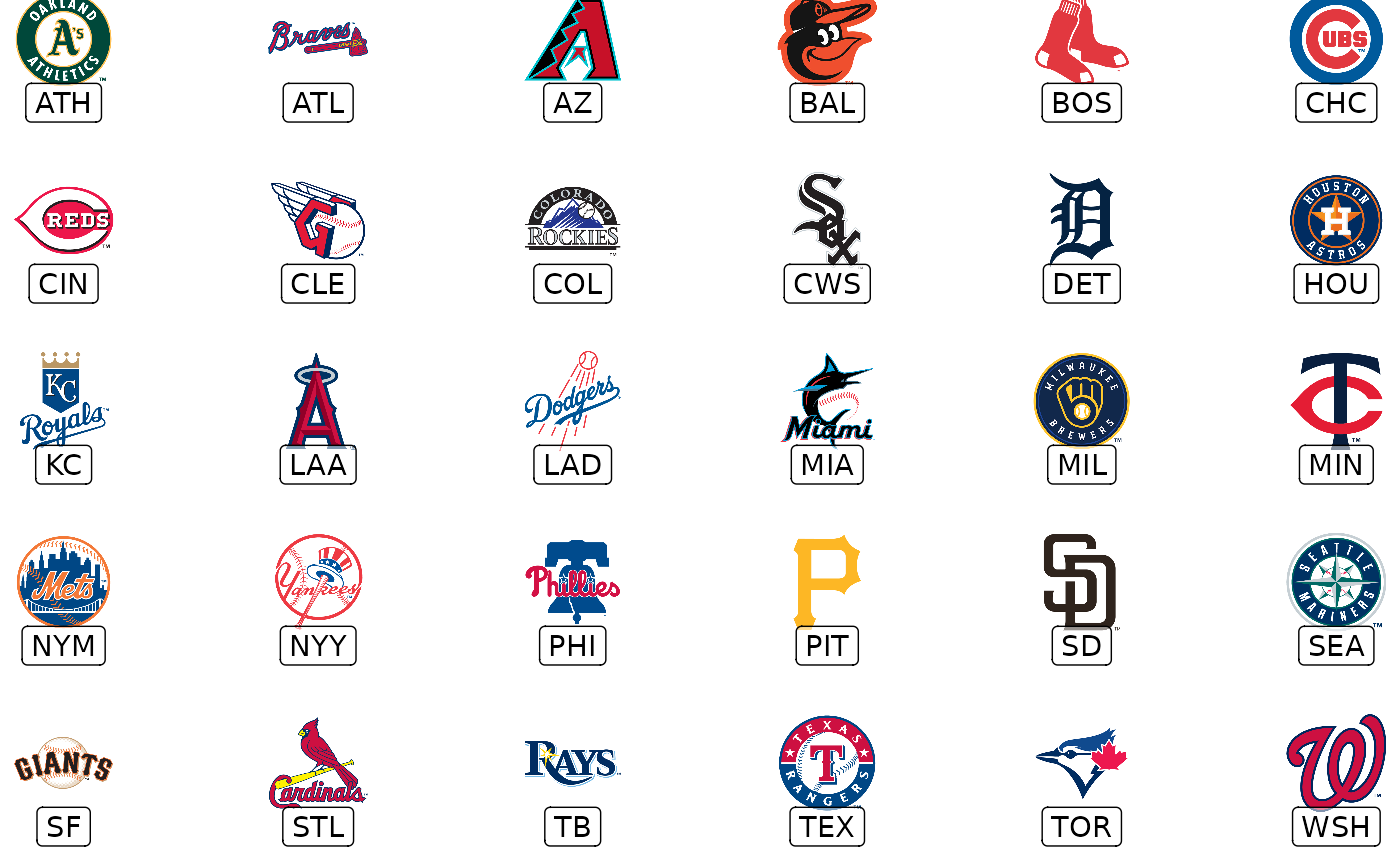# apply alpha via an aesthetic from inside the dataset df
# please note that you have to add scale_alpha_identity() to use the alpha
ggplot(df, aes(x = a, y = b)) +
geom_mlb_scoreboard_logos(aes(team_abbr = teams, alpha = alpha), width = 0.075) +
geom_label(aes(label = teams), nudge_y = -0.35, alpha = 0.5) +
scale_alpha_identity() +
theme_void()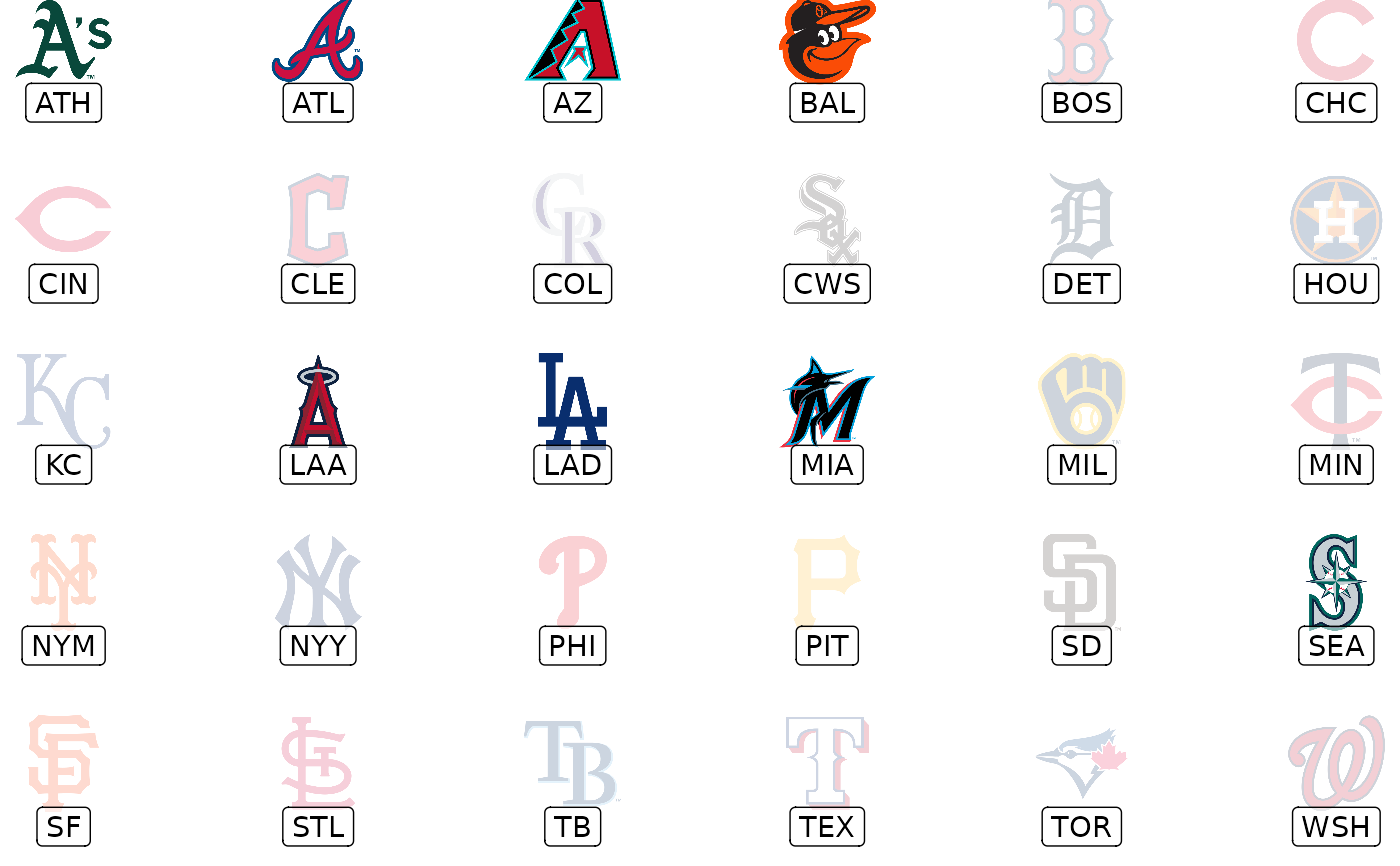# apply alpha and colour via an aesthetic from inside the dataset df
# please note that you have to add scale_alpha_identity() as well as
# scale_colour_identity() to use the alpha and colour values in your dataset!
ggplot(df, aes(x = a, y = b)) +
geom_mlb_logos(aes(team_abbr = teams, alpha = alpha, colour = colour), width = 0.075) +
geom_label(aes(label = teams), nudge_y = -0.35, alpha = 0.5) +
scale_alpha_identity() +
scale_colour_identity() +
theme_void()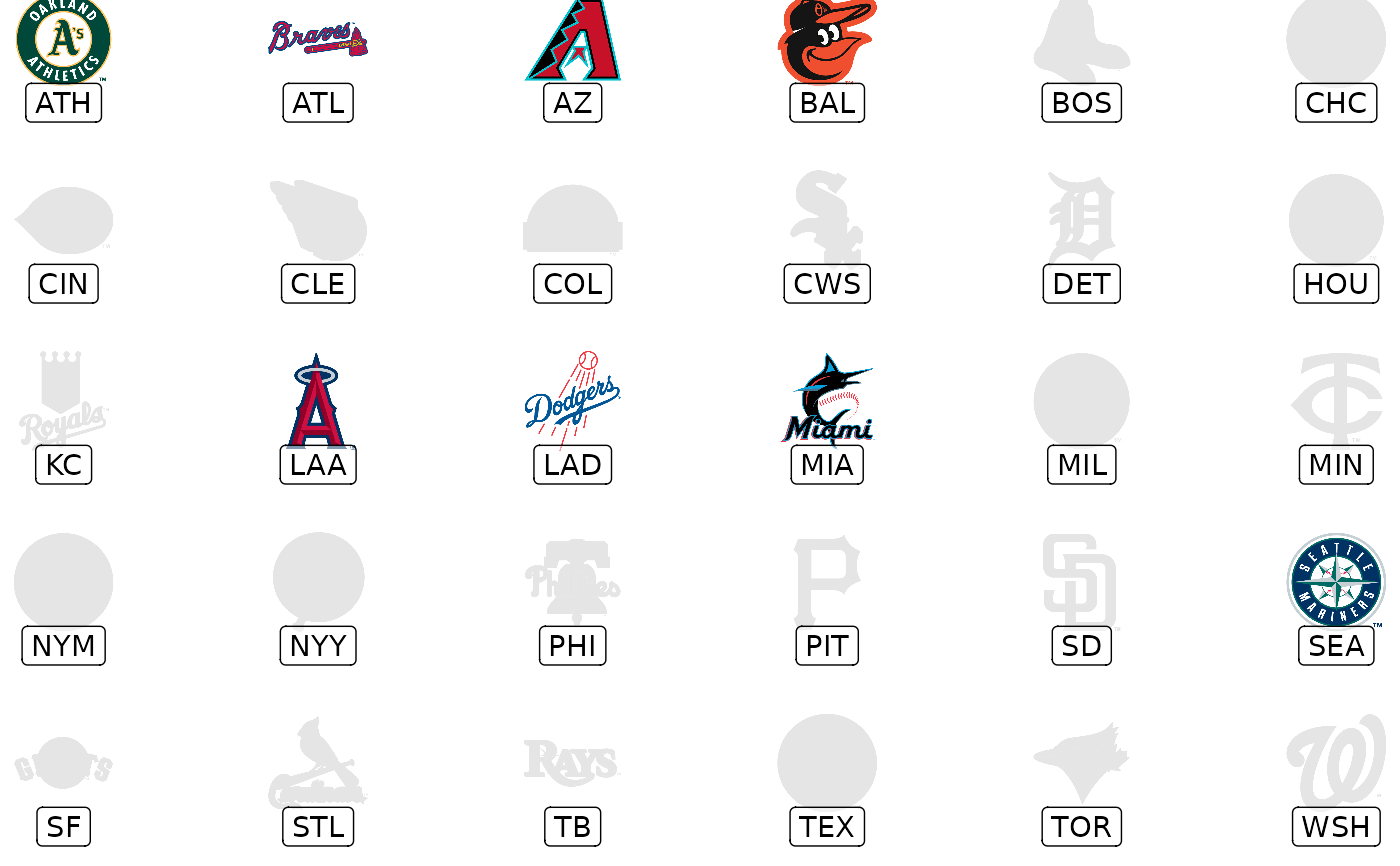# apply alpha as constant for all logos
ggplot(df, aes(x = a, y = b)) +
geom_mlb_scoreboard_logos(aes(team_abbr = teams), width = 0.075, alpha = 0.6) +
geom_label(aes(label = teams), nudge_y = -0.35, alpha = 0.5) +
theme_void()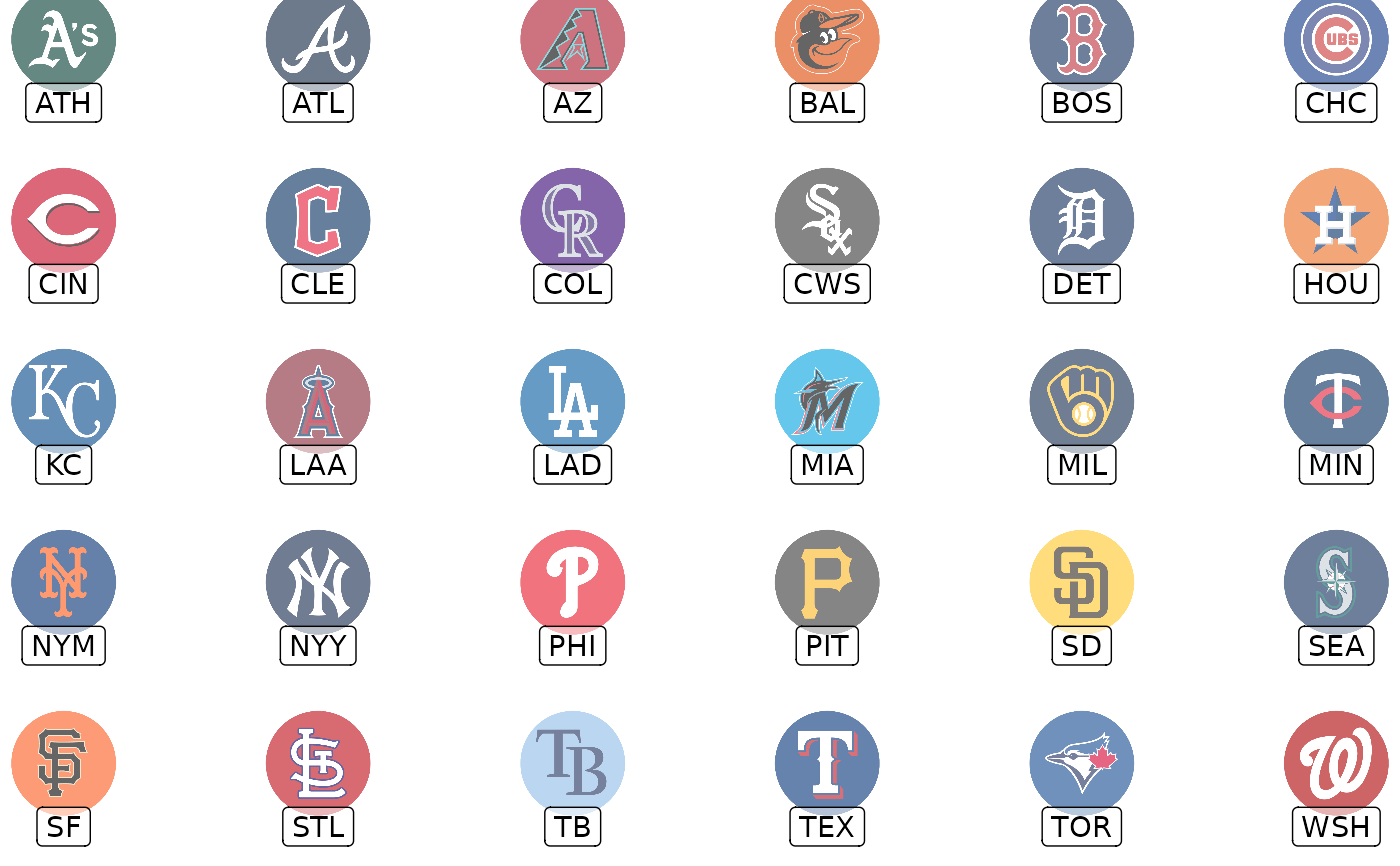# it's also possible to plot league logos
league <- data.frame(a = 1:3, b = 0, teams = c("AL", "NL", "MLB"))
ggplot(league, aes(x = a, y = b)) +
geom_mlb_logos(aes(team_abbr = teams), width = 0.3) +
geom_label(aes(label = teams), nudge_y = -0.4, alpha = 0.5) +
coord_cartesian(xlim = c(0.5,3.5), ylim = c(-0.75,.75)) +
theme_void()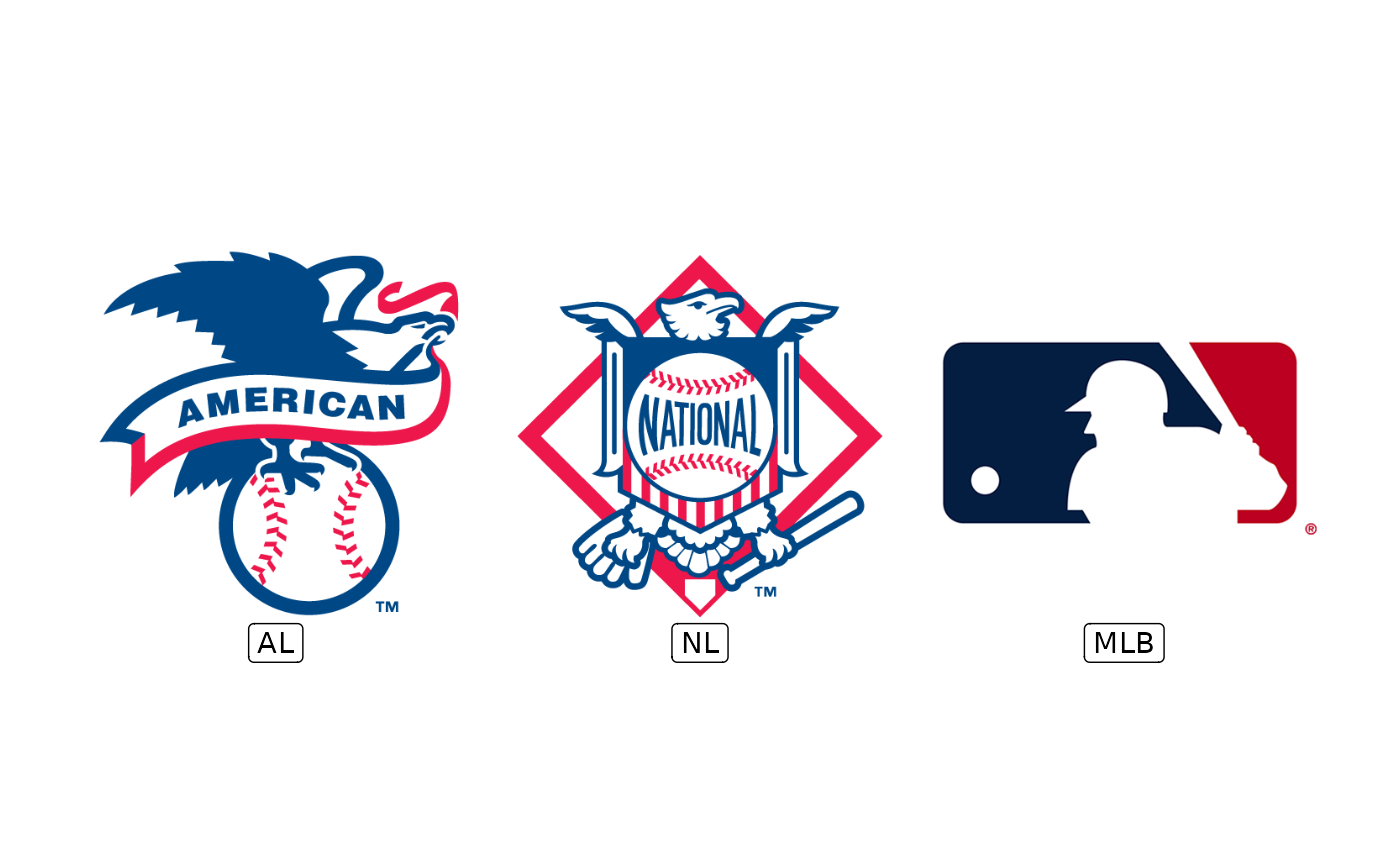# }